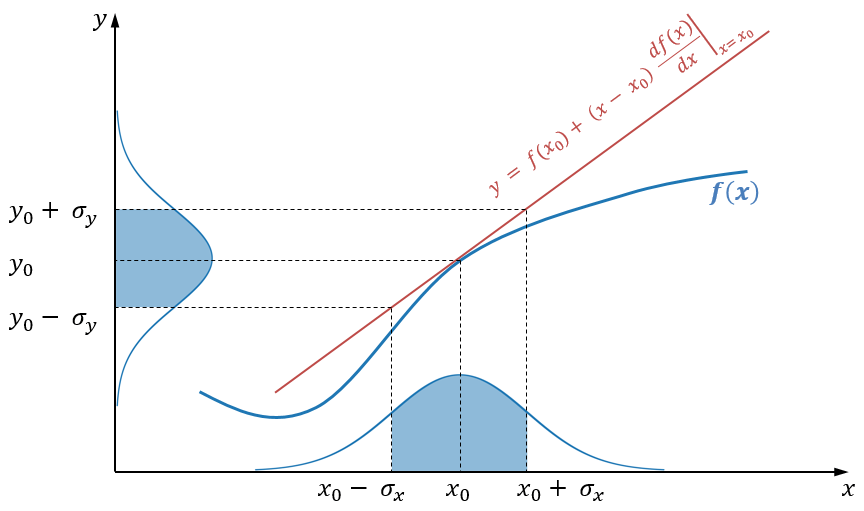﻿ Extended Kalman Filter

# Extended Kalman Filter

The Extended Kalman Filter has emerged from NASA Dynamic Analysis Branch research, led by Dr. Schmidt.

The main idea behind the EKF is a linearization of the dynamic model at the working point.

This chapter includes a detailed explanation of the concept and two numerical examples.

## Analytic linearization

The EKF performs analytic linearization of the model at each point in time. The following figure exemplifies a one-dimensional case. We want to find a tangent line for a point $$x = x_{0}$$. Using the tangent line, we can project the uncertainty to the y axis and keep its' shape Gaussian.